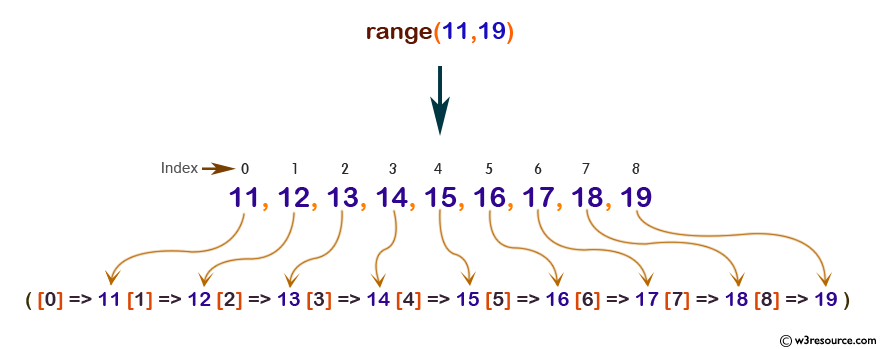# PHP: range() function

## PHP: Create an array containing a range of elements

The range() function used to create an array containing a range of elements.

Version:

(PHP 4 and above)

Syntax:

`range(low_value, high_value, step)`

Parameters:

Name Description Required /
Optional
Type
low_value Specify the lowest value. Required Mixed*
high_value Specify the highest value. Required Mixed*
step It is used as increment between elements. The default value is 1. Optional Integer/Float

*Mixed: Mixed indicates multiple (but not necessarily all) types.

Return value: An array.

Value Type : Array

Example:

``````<?php
\$number_list = range(11,19);
print_r (\$number_list);
echo "<br /> ";
\$number_list_step = range(11,19,2);
print_r (\$number_list_step);
echo "<br /> ";
\$letter_list = range("u","z");
print_r (\$letter_list);
?>
``````

Output:

```Array (  => 11  => 12  => 13  => 14  => 15  => 16  => 17  => 18  => 19  )
Array  (  => 11  => 13  => 15  => 17  => 19)
Array  (  => u  => v  => w  => x  => y  => z)
```

Pictorial Presentation:View the example in the browser

Practice here online :

PHP Function Reference

Previous: prev
Next: reset

﻿

## PHP: Tips of the Day

PHP: Extract numbers from a string

```\$str = 'In My Cart : 11 12 items';
preg_match_all('!\d+!', \$str, \$matches);
print_r(\$matches);
```